# Copper Removal From Paper Mill Wastewater Using Polymer Nanofiber

Document Type : Original Research Paper

Authors

1 Department of Chemical Engineering, Islamic Azad University, Ayatollah Amoli Branch, Amol, Iran

2 Department of Chemistry, Faculty of Pharmaceutical Chemistry, Tehran Medical Sciences, Islamic Azad University, Tehran, Iran

Abstract

The aim of this research work is to investigate sorption characteristic of synthesized polypyrrole / polyaniline (PPy / PANI) nano fiber for Cu (II) removal from paper mill wastewater. The sorption is carried out by batch method. The structural characterization of this sorbent was performed by SEM and FTIR analysis. The optimum conditions of copper sorption from paper mill wastewater onto PPy / PANI were found to be: pH=6, contact time 12 min and 0.4 g of sorbent in 100 ml in wastewater. The kinetic studies showed that the sorption process was well described by the pseudo second order kinetic model. Also, Langmuir isotherm provided the best fit to the equilibrium data. Also the effect of temperature on the process was found that the temperature has positive effect on the process. Thermodynamic parameters showed that the sorption of copper onto nano fiber were feasible, spontaneous and endothermic under studied conditions.

Keywords

#### Full Text

INTRODUCTION

The cellulose and paper industry is an almost global, being present in most developed and developing countries and is one of the most important environmental concerns . Heavy metals come from several industries such as mining and smelting, paper industry, industrial activities, municipal wastes, the printing, and photographic industries and sewage sludge [2, 3]. Although copper is one of the most toxic heavy metals, it has drawn much attention due to its toxicity and impact on public health and sometimes death, particularly in children [3-5]. Among several methods for heavy metals removal from solutions such as evaporation, electroplating, precipitation, ion exchange and membrane separation, adsorption proves to be an efficient and cost-effective method strongly recommended for low concentration, Many sorbents such as mesoporous silicates, Polyethyleneimine Methylenephosphonic acid , biomaterials , fiber membranes, ion exchange  and low-cost natural materials wereapplied for heavy metal sorption from solutions.

In recent decades, lots of literature have studied the use of conducting polymers such as polyaniline (PANI) and polypyrrole (PPy) for environmental remediation. Conducting polymer can lead to finding applications in several fields such as microelectronics, composite materials, optics, and biosensors  and as adsorbent [14, 15]. The ion exchange capacities of conducting polymers were found to depend on the polymerization conditions, the type and size of the dopants incorporated during the polymerization process as well as on the ions present in the electrolyte solution, the polymer thickness and aging of the polymer . Esfandian et al  have used polyaniline and polypyrrole composites for paper mill wastewater treatment. Also, the adsorbents were used as effective adsorbents for the removal of heavy metals, anions, color, and COD (chemical oxygen demand) from mill paper wastewater. In another study, an attempt was made to study the possibility of using polypyrrole composite on the perlite zeolite surface for removal of copper from wood and paper factories wastewater . Polyacrylamide (PAM) in polymer induced flocculation was also used as a sorbent for treatment of pulp and paper mill wastewater. The effectiveness of the polyacrylamides was measured based on the reduction of turbidity, the removal of total suspended solids (TSS), and the reduction of chemical oxygen demand (COD) . The aim of this studywas Cu (II) sorption by polymer nano fiber from industrial wastewater. The effects of nano fiber dosage, contact time, temperature, and pH value were investigated.Also sorptionkinetics, isotherms,thermodynamic properties of the process was obtained.

Materials and methods

Materials

Aniline (Merck) and pyrrole (Merck) were purified by vacuum distillation and stored in the refrigerator before use. The solution of Cu(II) was prepared from paper mill wastewater. The pH of the solution was monitored by adding 0.5 M HCl and 0.5 M NaOH solution as per required pH value. The FeCl3 was purchased from Merck.
Table 1shows the characteristics of the wastewater (paper mill factory in Sari, Iran).

Instrumentation

In this research, to study the nanofiber surface, the Scanning Electron Microscope (SEM) model S3400, Hitachi, Japan was used. Using a sputter coater, the sample was coated with gold and palladium. They are conductive materials to improve the quality of image. The coating thickness and the density were 30.00 nm and 19.32 g/cm3, respectively. The nanofiber infrared spectra (IR) were provided by FTIR Spectrometer (Shimadzu 4100) in order to form the nanofiber functional groups. Using a spectrometer and KBr pellets, spectra were collected. In each case, using mortar and pestle, 1.0 mg of dried DAS and 100 mg of KBr were homogenized and later pressed into a transparent tablet at 200 kgf/cm2 for 5 min. The pellets’ characterization was done using the FTIR spectrometer in the transmittance (%) mode with a scan resolution of 4 cm− 1 in the range of 3900–500 cm− 1.

The sorption experiments were done in ±25 ºc and the pH of the solution was regulated by pH-meter and also by using HCl and NaOH solutions. In each step, the exact amount of the adsorbent was added to the samples and was then mixed up together with the rotating speed of 400 rpm (by jartest machine made by Zaghshimi Company, Iran). At the end of the predetermined time intervals, the sorbent was filtered and the filtrate was analyzed for the remaining copper concentration in the sample using atomic absorption spectrophotometer according to the procedure reported in APHA, AWWA standard methods for an examination of water and wastewater(APHA and AWWA, 2005). All experiments were carried out twice and the adsorbed concentrations given were the means of duplicate experimental results. The average data was reported. In all experiments, sorbent was in the form of powder. The efficiency of Cu(II), % Removal, were calculated as: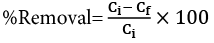(1)

Where Ci is the initial concentration (mg L−1) and Cf is the final concentration (mg L−1). q is the amount of metal adsorbed per the specific amount of adsorbent (mg g-1). The sorption capacity at time t, qt (mg g-1) was obtained as follows: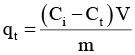(2)

Where Ci and C(mg L-1) are liquid-phase concentrations of solutes at initial and a given time t, respectively. V is the volume of the solution and m is the mass of nanofiber (g). The amount of adsorption at equilibrium, qe was given by: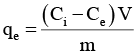(3)

Where C(mg L-1) was the ion concentration at equilibrium.

Synthesis of the PPy-PANI nanofibers

PPy-PANI nanofibers were synthesized using in situ simultaneous polymerizations of Py and ANI monomers in presence of FeCl3oxidant at room temperature. In a typical polymerization process (solution polymerization), 6 g of FeCl3 was dissolved in 80 mL of distilled water in a 250 ml conical flask. A mixture (0.8 mL) of PPy and ANI monomers (0.4 mL of each) was added dropwise into the oxidant solution under stirring. The used amount of oxidant has already optimized in our earlier studies . The reaction mixture was stirred for 5 min. Then the polymerization reaction was allowed to proceed without stirring for 6 h. After that, 10 mL of acetone was added to the reaction mixture to stop the reaction. The PPy-PANI nanofibers formed were filtered and washed with distilled water until the filtrate became colorless and thereafter washed with acetone to remove the oligomers . The nanofibers were then dried at 80 oC for 6 h under vacuum until the total mass became constant. The total weight of the PPy-PANI nanofibers was 0.65 g determined by gravimetry.

RESULTS AND DISCUSSION

Fig. 1 shows the morphology of polymer nanofiber. As can be seen, the spherical nano-sized particle has been formed with an average size of 25 nm. The polymer nanofiber structure has come under scrutiny using the FTIR technique and is shown in Fig.2. The characteristic IR peaks at 1513, 1430, 1082, and 957–825 cm−1 are attributed to the pyrrole ring stretching, conjugated C-N stretching, C-H stretching vibration, and C-H deformation, respectively . The main characteristic peaks for PANI homopolymer are assigned as follows: the bands at 1568and 1486 cm−1 correspond to quinine and benzene stretching ring deformation and the stretching bands at 1293, 1137 belong to C-N and N=Q=N (Q denotes the quinoid rings) . In the case of the PPy-PANI nanofibers, the observed IR peaks at 1541, 1447, 1039, and 930–837 cm−1 and 1568, 1490, 1276, 1128 cm−1 confirm the presence of both PPy and PANI polymeric moieties in the synthesized nanofibers.

Effect of pH

The effect of pH of the solution on Cu(II) sorption by polymer nanofiber from paper mill wastewater was investigated in an initial pH range of 2 to 6. Experiments were carried out with 100 mL of wastewater solution containing 1 g of the sorbent at different pHs, at 20 oC to study the effect of initial pH on the efficiency of the copper sorption (Fig.3). As can be seen from Fig. 3, increasing the pH leads to an increase in the Cu(II) adsorption amount. This increase gradually reached a maximum value sharply at pH 6. Working over pH=6.0 was avoided to prevent the possible precipitation of copper. It can be deduced that, at low pH values, the concentration of H+ ions increases, and thus an intense competition occurs between H+ ions and Cu(II) ions for sorption on binding sites of polymer nanofiber. As a result, H+ ions could either occupy the binding sites or protonate functional groups on polymer nanofiber, i.e. metal-binding sites. Because of protonation, the amount of positively charged sites increases, the corresponding electrostatic repulsion restricts the adsorption of positively charged copper ions. Thus, the sorption capacity of copper ions reduced at pH 2.0. On the other hand, as the pH values increased, the negative charge density on the surface of polymer nanofiber also increased due to deprotonation of the metal-binding sites. This in turn enhances the affinity toward positively charged Cu(II) ions and leads to the improved adsorption of metal ions [23, 24]. Thus, all future sorption experiments in this research were conducted at an initial pH value of 6.0.

Effect of contact time

The effect of contact time on the sorption of Cu(II) was studied by polymer nanofiber(1 g in 100 mL ) were with an initial copper concentration in paper mill wastewater, pH 6, and temperature of 20 oC (Fig. 4). The amount of Cu(II)sorption is increased by every increase in contact time. Under the above conditions, Fig. 4 shows that the adsorption amount reaches the maximum value after 12 min and a subsequent little change of sorption occurs (i.e, remains constant thereafter). In fact, one can mention that the time of every part of the system and the respective sensitivity to deviation, have a direct and/or indirect effect on all costs and yields. It should be noted that the contact time in the absorption process is considered a parameter of equilibrium. This signifies that the variable was optimized and the subsequent increase in the point of equilibrium has no effect on the absorption process. Therefore, future studies should be conducted on the variables that are of high importance and necessity[25, 26].

Kinetics of sorption

Morris–Weber Kinetic model

To investigate the change in the concentration of sorbate onto sorbent with shaking time, the kinetic data of Cu(II) ions sorption onto beach sand was subjected to Morris–Weber Eq. (4) :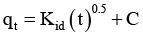(4)

where qt is the sorbed concentration of Cu(II) ions at the time ‘t’. The plot of qt versus t0.5 is given in Fig.5 and found to be linear with a correlation factor of 0.9941. The value of rate constant of intraparticle transport, Kid, calculated from the slope of the linear plot is 1.4753min-1. Internal particle diffusion may involve pore and/or surface diffusion. The intraparticle diffusion plots show multi-linearity in the process indicating that three steps are operational. The first stage can be attributed to the diffusion of adsorbate through the solution to the external surface of the adsorbent or the boundary surface diffusion of the sorbate molecules. The second stage describes the gradual sorption, where intraparticle diffusion is rate-limiting, and the third stage is attributed to the final equilibrium due to extremely low sorbate concentration left in solution and the reduction of interior active sites. As can be seen, only one linear portion appeared in Fig.5, which might be due to intraparticle diffusion effects. This further indicated that the intraparticle diffusion was only the rate-controlling step rather than the other processes. High values of r2 were obtained for copper sorption, suggesting that intraparticle diffusion was involved in the adsorption of copper ions by S. bevanom under the experimental conditions. However, the regression lines did not pass through the origin of the plot, and a positive intercept was observed, indicating that another process was also involved in the adsorption of metal ion onto S. bevanom. The three stages in the plot suggest that the sorption process occurs by surface adsorption and intraparticle diffusion.

Lagergren kinetic model

The pseudo-first-order of the sorption of Cu(II) ions onto polymer nanofiber was evaluated by treating the data to the following form of Lagergren rate expression (Eq. 5) , to determine the rate constant of sorption for Cu(II) ions–nanofiber system.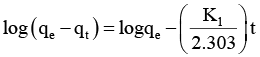(5)

where qe is the sorbed concentration at equilibrium and k is the first-order rate constant. The linear plot of log(qe−qt) against time ‘t’(Fig.6)demonstrates the applicability of the above equation for copper ions sorption onto polymer nanofiber. The rate constant k1= 0.4795 min−1 was calculated from the slope of the straight line with a correlation factor of 0.7611.

Pseudo-second order kinetic model

The sorption of copper ions onto sorbent following pseudo-second-order kinetics can be represented as Eq. (6)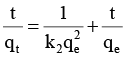(6)

where qt and qare the amounts of ion adsorbed at time “t” and equilibrium (mg.g−1) and k2( g.mg−1min−1) is the pseudo-second-order rate constant for the adsorption process.Fig.7 depicts the linear plots of the pseudo-second-order model. The rate constant k2 =0.0487(g.mg−1min−1) was calculated from the slope of the straight line using the correlation factor of 0. 9995.

The kinetic data showed that the adsorption process was controlled by the pseudo-second-order equation. Based on the above assumption, the Cu(II) uptake process occurs due to chemisorptions. The fact that the rate-limiting step might be chemisorptions involving valence forces through sharing or exchanging of electrons between adsorbent and adsorbate forms the assumption of the pseudo-second-order kinetic model. The dimethyl phthalate molecule’s behavior in the system is strongly dependent upon the concentration and the properties of other species, pH of the solution, physical and chemical properties of both the adsorbent and adsorbate. The adsorption kinetics form and its related coefficients in the system were influenced by both the interaction and competition effects among the Cu(II).

The effect of polymer nanofiber dose was investigated at the dose between 0.o5 g and 0.5 g in 100 mL of paper mill wastewater. The experiments were performed at 20°C, pH 6, contact time 12 min in 100ml solution. It was found out that increasing the sorbent dose increases the amount of adsorbed Cu(II)(Fig. 8). Shortly after, it was discovered that the availability of larger surface area and more adsorption sites increases with every increase in the adsorbent dose. Increasing the dried activated sludge at higher concentrations of sorbate did not cause the equilibrium uptake to increase significantly. To fit different isotherm models, i.e., Langmuir, Freundlich, and Dubinin-Randkovich (D-R), the data of Fig. 8 were used.

The isotherm model

The adsorption isotherm model is based upon the hypothesis saying that all adsorption sites are equivalent and their status does not depend on whether the neighboring sites are occupied or not. Isotherms describe the relationship between metal content of a solution and the amount of metal adsorbed on a specific adsorbent at a constant temperature.

The Langmuir isotherm model

The Langmuir model is valid and applicable for adsorptions onto a surface containing a limited number of identical sorption sites. This isotherm is often offered in the form of the following equation Eq. (7)[30-32]: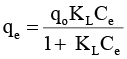(7)

Where qe is the amount of metal adsorbed per specific amount of adsorbent (mg g-1), Ce, the equilibrium concentration of the solution (mg L-1), and q0, the maximum amount of adsorption metal ions (mg g-1). The Langmuir equation can be rearranged to linear form for the convenience of plotting and determining the Langmuir constants (KL) as below. The values of qo and KL can be determined from the linear plot of Ce/qe versus Ce: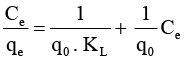(8)

Langmuir equation constants can be rearranged to linear types given in Table 2. Fig.9 shows plots of Ce/qe versus Ce for adsorption isotherms. As can be seen, Langmuir shows acceptable fitting by data.

The Freundlich isotherm model

While Langmuir isotherm assumes that enthalpy of adsorption is independent of the amount adsorbed, the empirical Freundlich equation, based on sorption on the heterogeneous surface, can be derived assuming a logarithmic decrease in the enthalpy of adsorption with the increase in the fraction of occupied sites. The Freundlich equation is purely empirical based on sorption on the heterogeneous surface and is given by Eq. (9):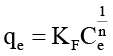(9)

Where Kand (1/n) are the Freundlich constants related to adsorption capacity and sorption intensity, respectively. Equilibrium constants evaluated from the intercept and the slope, respectively, of the linear plot of log qversus log Ce based on experimental data. The Freundlich equation can be linearized in logarithmic form for the determination of the Freundlich constants as Eq. (10):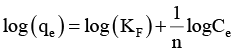(10)

The slope and the intercept correspond to (1/n) and KF, respectively. It was revealed that the plot of log qand log Ce yields a straight line (Fig.10). The Freundlich sorption constants and the regression correlation coefficient are given in Table 2.

The Dubinin–Radushkevick (D–R) [33-35]isotherm was used to determine the nature of the adsorption process viz. physisorption or chemisorption. The linear form of this model is expressed by Eq. (11):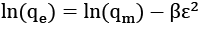(11)

Where qe is the amount of Cu(II) adsorbed per unit dosage of the adsorbent (mg/g), qm the monolayer capacity and β is the activity coefficient related to mean sorption energy and ε is the Polanyi potential described as Eq. (12):

ε=RT ln [١+ (١/Ce)] (12)

From the plots of lnqe versus ε2(Fig.11), the values of βand qm were determined by the slope and intercept of the linear plot. The statistical results along with the isotherm constants are given in Table 2. As our results show, adsorption of Cu (II) by nanofiber can be fitted using Freundlich equations.

Effect of Temperature on the copper sorption

The sorption studies were conducted at 20–40oC, pH 6, and adsorbent dosage of 0.4 g in a 100 mL wastewater solution to examine the thermodynamics of adsorption. The equilibrium contact time for adsorption was kept constant at 12 min. The adsorption percentage increased with the rise in temperature from 20 to 40oC. Results suggested that the adsorption process has an endothermic nature. Table 3 explains the effect of temperature on the removal efficiency. To determine the changes in Gibbs free energy (ΔG), the heat of adsorption (ΔH), and entropy (ΔS) of the adsorption of copper from wastewater, the Table 3 data were used.

Effect of Temperature on Thermodynamics Parameter on Cu(II)

Different thermodynamic parameters such as the enthalpy change ΔH, free energy change ΔG, and entropy change ΔS were calculated using the equations (13-15) to study the thermodynamics of adsorption of Cu(II) on polymer nanofiber. Using the following equations, the thermodynamic parameters ΔH, ΔS, and ΔG for Cu(II) on a polymer nanofiber system were calculated as follows[31, 36]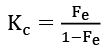(13)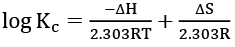(14)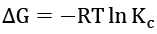(15)

where Fe is the fraction of Cu(II) sorbed at equilibrium. The values of these parameters are given in Table 4. It shows that the enthalpy change ΔH is positive (endothermic) because of the increase in adsorption on a successive increase in temperature. The negative ΔG values revealed that the nature of sorption is thermodynamically feasible and spontaneous. The positive value of ΔS indicates the increased randomness at the solid-solution interface during the fixation of the ion on the active sites of the sorbent.

CONCLUSION

The polymer nanofiber showed considerable potential for the removal of copper from the paper mill wastewater. The conditions of sorption are as follows: a sorbent dose of 0.4 g in 100 ml of solution, the contact time of 12 min, and a pH 6. The kinetic data shows that the adsorption process is controlled by the pseudo-second-order equation. The results obtained from this study were given by the theoretical Freundlich. Thermodynamic studies are indicative of a negative ΔG and positive ΔSand ΔH. Results showed that sorption has an endothermic nature. The negative ΔG values suggested that the sorption has a thermodynamically feasible and spontaneous nature. The positive value of ΔS indicates that there is increased randomness at the solid-solution interface during the ﬁxation of the ion on the sites of the sorbent.

CONFLICTS OF INTEREST

There are no conflicts to declare.